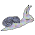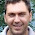## Tuesday, October 30, 2012

### DINEOF (Data Interpolating Empirical Orthogonal Functions)

I finally got around to reproducing the DINEOF method (Beckers and Rixon, 2003) for optimizing EOF analysis on gappy data fields - it is especially useful for remote sensing data where cloud cover can result in large gaps in data. Their paper gives a nice overview of some of the various methods that have been used for such data sets. One of these approaches, which I have written about before,  involves deriving EOFs from a covariance matrix as calculated from available data. Unfortunately, as the author's point out, such covariance matrices are no longer positive-definite, which can lead to several problems. The DINEOF method seems to overcome several of these issues.

DINEOF is a procedure fills gaps by iteratively decomposing the data field via Singular Value Decomposition (SVD) until a best solution is found as compared to a subset of reference values (non-gaps). This is done by progressively including more EOFs in the reconstruction of the gappy locations until minimization of error converges. The authors first demonstrate the method using a synthetic example (e.g. above figure), where: 1) a data set is created, 2) noise is added (based on a noise / signal setting), 3) gaps are created (based on a fraction of gappiness setting), and finally the gaps are interpolated via the DINEOF method. The figure below shows a record of the iterations performed to fill in the gaps with increasing number of EOFs. An optimized solution occurred using the leading 6 EOFs - the addition of a 7th EOF did not improve the root mean squared error (RMS).

The synthetic example was based on 9 combined signals. Below we see a fit of the EOFs of the "true" field (black points) and the optimized solution of the gappy data (red lines). Again, only 5 EOFs seem to represent a real signal. This is a result of the addition of the noise - a non-noise version usually iterates until the full 9 EOF modes.

Here is an animation of the optimization process:

Below is a version of DINEOF for R and a script to reproduce the example. Additional information on DINEOF can be found here. This code is my interpretation of the DINEOF procedure - use at your own risk! Take care when using the default setting for ref.pos (positions to zero out and use as reference points in the conversion). As is, this samples 30 OR 1% of the values, whichever is bigger.

1. The dineof() function has now been optimized through the use of partial Singular Value Decomposition (svd) via the irlba package. The function irlba() now replaces svd(). This package will need to be loaded in order to run dineof. This speeds up the algorithm substantially.
2. A comparison of EOF methods for gappy data can be found here: http://menugget.blogspot.de/2014/09/pca-eof-for-data-with-missing-values.html
3. The function can be downloaded as part of the R package "sinkr": https://github.com/marchtaylor/sinkr

My version of the DINEOF procedure:
```#This function is based on the DINEOF (Data Interpolating Empirical Orthogonal Functions)
#procedure described by Beckers and Rixon (2003).
#
#The arguments are:
#Xo - a gappy data field
#nu - a maximum number of EOFs to iterate (leave equalling "NULL" if algorithm shold proceed until convergence)
#ref.pos - a vector of non-gap reference positions by which errors will be assessed via root mean squared error ("RMS").
#If ref.pos = NULL, then either 30 or 1% of the non-gap values (which ever is larger) will be sampled at random.
#delta.rms - is the threshold for RMS convergence.
#
#The results object includes:
#Xa - the data field with interpolated values (via EOF reconstruction) included.
#n.eof - the number of EOFs used in the final solution
#RMS - a vector of the RMS values from the iteration
#NEOF - a vector of the number of EOFs used at each iteration
#
#Beckers, Jean-Marie, and M. Rixen. "EOF Calculations and Data Filling from Incomplete Oceanographic Datasets."
#Journal of Atmospheric and Oceanic Technology 20.12 (2003): 1839-1856.
#
#4.0: Incorporates irlba algorithm and is only the dineof procedure
#
dineof <- function(Xo, n.max=NULL, ref.pos=NULL, delta.rms=1e-5){

library(irlba)

if(is.null(n.max)){
n.max=dim(Xo)
}

na.true <- which(is.na(Xo))
na.false <- which(!is.na(Xo))
if(is.null(ref.pos)) ref.pos <- sample(na.false, max(30, 0.01*length(na.false)))

Xa <- replace(Xo, c(ref.pos, na.true), 0)
rms.prev <- Inf
rms.now <- sqrt(mean((Xa[ref.pos] - Xo[ref.pos])^2))
n.eof <- 1
RMS <- rms.now
NEOF <- n.eof
Xa.best <- Xa
n.eof.best <- n.eof
while(rms.prev - rms.now > delta.rms & n.max > n.eof){ #loop for increasing number of eofs
while(rms.prev - rms.now > delta.rms){ #loop for replacement
rms.prev <- rms.now
SVDi <- irlba(Xa, nu=n.eof, nv=n.eof)
RECi <- as.matrix(SVDi\$u[,seq(n.eof)]) %*% as.matrix(diag(SVDi\$d[seq(n.eof)], n.eof, n.eof)) %*% t(as.matrix(SVDi\$v[,seq(n.eof)]))
Xa[c(ref.pos, na.true)] <- RECi[c(ref.pos, na.true)]
rms.now <- sqrt(mean((Xa[ref.pos] - Xo[ref.pos])^2))
print(paste(n.eof, "EOF", "; RMS =", round(rms.now, 8)))
RMS <- c(RMS, rms.now)
NEOF <- c(NEOF, n.eof)
gc()
if(rms.now == min(RMS)) {
Xa.best <- Xa
n.eof.best <- n.eof
}
}
n.eof <- n.eof + 1
rms.prev <- rms.now
SVDi <- irlba(Xa, nu=n.eof, nv=n.eof)
RECi <- as.matrix(SVDi\$u[,seq(n.eof)]) %*% as.matrix(diag(SVDi\$d[seq(n.eof)], n.eof, n.eof)) %*% t(as.matrix(SVDi\$v[,seq(n.eof)]))
Xa[c(ref.pos, na.true)] <- RECi[c(ref.pos, na.true)]
rms.now <- sqrt(mean((Xa[ref.pos] - Xo[ref.pos])^2))
print(paste(n.eof, "EOF", "; RMS =", round(rms.now, 8)))
RMS <- c(RMS, rms.now)
NEOF <- c(NEOF, n.eof)
gc()
if(rms.now == min(RMS)) {
Xa.best <- Xa
n.eof.best <- n.eof
}
}

Xa <- Xa.best
n.eof <- n.eof.best
rm(list=c("Xa.best", "n.eof.best", "SVDi", "RECi"))

Xa[ref.pos] <- Xo[ref.pos]

RESULT <- list(
Xa=Xa, n.eof=n.eof, RMS=RMS, NEOF=NEOF
)

RESULT
}```
Created by Pretty R at inside-R.org

To reproduce the example (follows that contained in the paper by Beckers and Rixon )
```#color palette
pal <- colorRampPalette(c("blue", "cyan", "yellow", "red"))

#Generate data
m=50
n=100
frac.gaps <- 0.5 # the fraction of data with NaNs
N.S.ratio <- 0.1 # the Noise to Signal ratio for adding noise to data

x <- (seq(m)*2*pi)/m
t <- (seq(n)*2*pi)/n

#True field
Xt <-
outer(sin(x), sin(t)) +
outer(sin(2.1*x), sin(2.1*t)) +
outer(sin(3.1*x), sin(3.1*t)) +
outer(tanh(x), cos(t)) +
outer(tanh(2*x), cos(2.1*t)) +
outer(tanh(4*x), cos(0.1*t)) +
outer(tanh(2.4*x), cos(1.1*t)) +
tanh(outer(x, t, FUN="+")) +
tanh(outer(x, 2*t, FUN="+"))

Xt <- t(Xt)
image(Xt, col=pal(100))

#Noise field
set.seed(1)
RAND <- matrix(runif(length(Xt), min=-1, max=1), nrow=nrow(Xt), ncol=ncol(Xt))
R <- RAND * N.S.ratio * Xt

#True field + Noise field
Xp <- Xt + R
image(Xp, col=pal(100))

#Observed field with gaps
set.seed(1)
gaps <- sample(seq(length(Xp)), frac.gaps*length(Xp))
Xo <- replace(Xp, gaps, NaN)
image(Xo, col=pal(100))

#
set.seed(1)
RES <- dineof(Xo)
Xa <- RES\$Xa

image(Xa, col=pal(100))

#plot of rms convergence
png("dineof_rms_convergence.png", width=5, height=4, units="in", res=400)
par(mar=c(5,5,3,5))
plot(RES\$RMS, xlab="Iteration", ylab="RMS", log="y", t="l", lwd=2)
par(new=TRUE)
plot(RES\$NEOF, xaxt="n", yaxt="n", xlab="", ylab="", t="l", lwd=2, col=2)
axis(4)
mtext("# EOFs", side=4, line=3, col=2)
dev.off()

#plot of fields
png("dineof_composite.png", width=8, height=8, units="in", res=400)
#x11(width=8, height=8)
ZLIM <- range(Xt, Xp, Xo, Xa, na.rm=TRUE)
par(mfrow=c(2,2), mar=c(3,3,3,1))
image(z=Xt, zlim=ZLIM, main="A) True", col=pal(100), xaxt="n", yaxt="n", xlab="", ylab="")
box()
mtext("t", side=1, line=0.5)
mtext("x", side=2, line=0.5)
image(z=Xp, zlim=ZLIM, main=paste("B) True + Noise (N/S = ", N.S.ratio, ")", sep=""), col=pal(100), xaxt="n", yaxt="n", xlab="", ylab="")
box()
mtext("t", side=1, line=0.5)
mtext("x", side=2, line=0.5)
box()
image(z=Xo, zlim=ZLIM, main=paste("C) Observed (", frac.gaps*100, " % gaps)", sep=""), col=pal(100), xaxt="n", yaxt="n", xlab="", ylab="")
mtext("t", side=1, line=0.5)
mtext("x", side=2, line=0.5)
image(z=Xa, zlim=ZLIM, main="D) Reconstruction", col=pal(100), xaxt="n", yaxt="n", xlab="", ylab="")
box()
mtext("t", side=1, line=0.5)
mtext("x", side=2, line=0.5)

dev.off()

#plot of EOF coefficients
St <- svd(Xt)
Sa <- svd(Xa)
png("dineof_EOF_coefficients.png", width=8, height=8, units="in", res=400)
#x11(width=8, height=8)
par(mfrow=c(3,3), mar=c(3,3,3,1))
for(i in seq(9)){
YLIM <- range(St\$u[,i], Sa\$u[,i])
plot(t, St\$u[,i], ylim=YLIM, xlab="", ylab="")
lines(t, Sa\$u[,i], col=2, lwd=2)
mtext(paste("EOF coeff.", i), side=3, line=0.5)
}
dev.off()```
Created by Pretty R at inside-R.org

References

1.Thanks for this - a very interesting article.

A simple method for dealing with gappy rasters, involving voter-model interpolation, was suggested by Clint Sprott a few years ago:

http://sprott.physics.wisc.edu/pubs/paper276.htm

It's only useful when the data are fractal-ish.

2.Thanks for the interesting reference Michael - Benoit Mandelbrot would have been proud of that application.

3.I think you forgot to define mu_Noise ?
but this is great stuff - thanks for sharing.

4.Thanks for your nice words and for noticing that missing part of the code - I have defined mu_Noise now in the example. Cheers

5.Correction - Noise is now implemented using a randomly generated matrix of uniform distribution (RAND), which is multiplied by the N/S ratio and the true field : R <- RAND * N.S.ratio * Xt. This is then added to the true field to derive the noisy field (Xp).

6.Very interesting! I have been waiting for this to happen in R. As a R beginner, how could I run this in R? Tried to copy your script and run in in R but could not get it running. Could you kindly help?

1.Thanks for your interest. I just copied the two sections of code into R and it runs fine. Without specifics as to what is not working, I'm unable to comment on how to fix it. Generally, you will need to load the dineof() function code first, and then run the example script to produce the analysis. Cheers

7."30 OR % of the values" (not 1%).
Thanks for sharing this great work.
Michael

1.Thanks for pointing out this error - I actually think the recommended number of reference values is >30 OR 1% with a large data set. So, I have now adjusted the default of the function rather than the blog post. Cheers, Marc

8.Great code, works well--thanks for putting this together. I have a question about interpolating when part of the grid includes land. How do you mask out pixels that you want to exclude from the interpolation?
-Nick

1.Thanks for your comments. You should simply remove those spatial locations (i.e. columns) from the matrix before performing dineof.

2.Hi Marc,

Thanks for the quick reply. I don't quite follow. Suppose I have a matrix that looks like this:

5 7 NA 8 3
2 6 5 NA 2
2 NA NA 10 3
4 6 XXX XXX XXX
1 XXX XXX 4 5

And I want to interpolate the NAs, but not the positions that have XXX. What should I do?

Thanks
Nick

3.The typical organization of the field matrix is to have spatial grids as columns and time as rows. Here is an example that filters out "land" grids (i.e. columns) from the slp dataset in the sinkr package:

library(sinkr)
data(slp)

Field <- slp\$field # only the values matrix
Land <- 200:231 # columns with land
Field2 <- Field[,-Land] # remove land grids
grid2 <- slp\$grid[-Land,]

plot(grid2, col=val2col(Field2[1000,], col=jetPal(20)), pch=".", cex=20)

9.This comment has been removed by the author.

10.Hey! I was looking for some way to deal with NAs in my data and just tried to apply your function dineof() on my data frame. But it keeps telling me:
Error in `[<-.data.frame`(`*tmp*`, list, value = 0) :
new columns would leave holes after existing columns

Do you know, what might be going wrong?

1.Sorry, I don't know what is causing this error. You may want to convert your data.frame to a matrix, and make sure that you do not have any non-numeric variables.